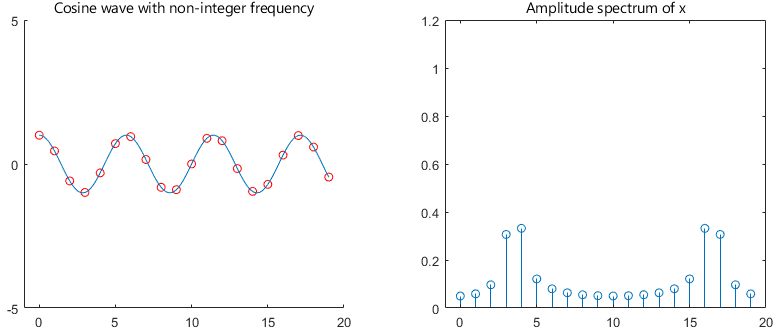StatLect

# Discrete Fourier transform of cosine and sine waves

The Discrete Fourier Transforms (DFTs) of cosine and sine waves have particularly simple analytical expressions.## The transform

Letbe anvector.

The Discrete Fourier Transform ofis anothervectorwhose entries satisfywhereis the imaginary unit.

We can use the DFT to obtain the frequency-domain representation## Properties of trigonometric functions and complex exponentials

To derive the DFT of the sine and cosine functions, we will use the following properties.

For any angle, we haveSince the period of the trigonometric functions is, we havefor any integerand any angle.

Finally, we have## DFT of a cosine wave with integer frequency

Let us start from a cosine wave:where.

The numberof cycles persamples (frequency) is assumed to be a positive integer less than.

The cosine wave can be written as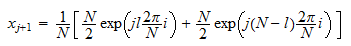which implies that its Discrete Fourier Transform isProof

We can write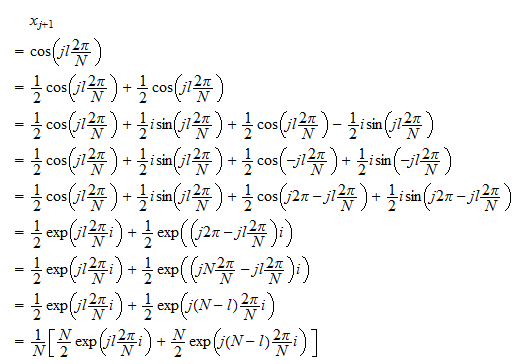which is a frequency-domain representation ofas a linear combination of periodic basis functions. The two basis functions belong to the set of basis functions used in the DFT. Since the representation of a vector as a linear combination of a basis is unique, the coefficients of the linear combination inside the square brackets must be the values of the discrete Fourier transform. They are all equal to, except those corresponding to the frequenciesand, which are equal toandrespectively.

## DFT of a sine wave with integer frequency

Consider the sine wave:where.

The numberof cycles persamples is again assumed to be a positive integer less than.

The sine wave can be written as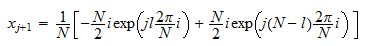which implies that the Discrete Fourier Transform isProof

We have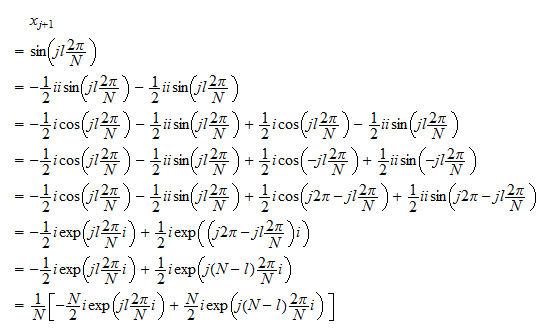which is a frequency-domain representation ofas a linear combination of DFT basis functions. Therefore, the coefficients of the linear combination inside the square brackets are the values of the DFT (see also the previous proof). They are all equal to, except those corresponding to the frequenciesand, which are equal toandrespectively.

## Linear combinations of sine and cosine waves

Since the DFT is a linear operator, we can use its linearity to easily derive the DFT of linear combinations of sine and cosine waves.

Example Letandbe positive integers, with. Let the entries ofbe defined byThen, the DFT ofis## Cosine with phase shift

Let us now analyze the case of a shifted cosine wave:where:

•;

•is a positive integer less than;

• the phasesatisfies.

The Discrete Fourier Transform is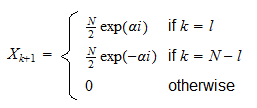Proof

We can derive the frequency-domain representation ofas follows: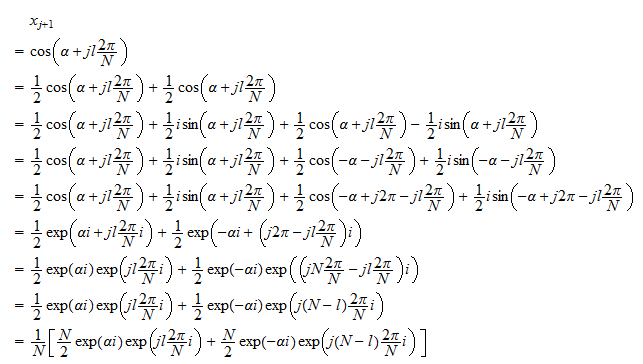As in the previous proof, we can easily read the values of the DFT from the frequency-domain representation: all the values of the DFT are equal to, except those corresponding to the frequenciesand, which are equal toandrespectively.

## Sine with phase shift

Similarly, we can analyze a shifted sine wave:where:

•;

•is a positive integer less than;

• the phasesatisfies.

The Discrete Fourier Transform is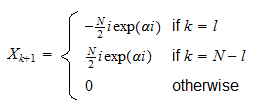Proof

We can derive the frequency-domain representation ofas follows: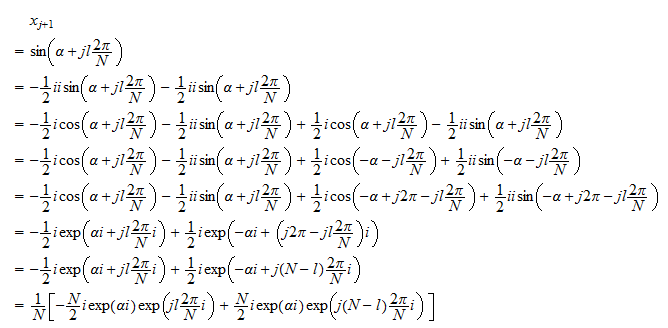As in the previous proofs, we can read the values of the DFT from the frequency-domain representation: all the values of the DFT are equal to, except those corresponding to the frequenciesand, which are equal toandrespectively.

## Spectral leakage

What happens whenorbut the frequency parameteris not an integer?

In other words, what happens when the sine or cosine waves have frequencies that do not belong to the set of analysis frequencies used to compute the DFT?

What happens is that the neat results presented above (DFT is zero everywhere except at the frequenciesand) are no longer valid.

Whenis not integer, the DFT can be non-zero everywhere, although it tends to be larger in magnitude around the frequenciesand. This phenomenon is known as spectral leakage.

### Example of spectral leakage

As an example, we set,and.

Then, we compute the DFTand its amplitude spectrumThe amplitude spectrum, displayed in the next plot, clearly shows that although the amplitudes are large around theandfrequencies, there is leakage to all the other frequencies.